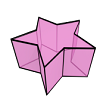# Modeling and manipulating spacetime objects in a true 4D model

## Authors

• Ken Arroyo Ohori
• Hugo Ledoux
• Jantien Stoter

## Keywords:

4D GIS, spacetime, spatiotemporal modeling, spatiotemporal operations, geometric modeling

## Abstract

The concept of spacetime has long been used in physics to refer to models that integrate 3D space and time as a single 4D continuum. We argue in this paper that it is also advantageous to use this concept in a practical geographic context by realizing a true 4D model, where time is modeled and implemented as a dimension in the same manner as the three spatial dimensions. Within this paper we focus on 4D vector objects, which can be implemented using dimension-independent data structures such as generalized maps. A 4D vector model allows us to create and manipulate models with actual 4D objects and the topological relationships connecting them, all of which have a geometric interpretation and can be constructed, modified, and queried. In this paper we discuss where such a 4D model fits with respect to other spatiotemporal modeling approaches, and we show concretely how higher-dimensional modeling can be used to represent such 4D objects and topological relationships. In addition, we explain how the 4D objects in such a system can be created and manipulated using a small set of implementable operations, which use simple 3D space and 1D time inputs for intuitiveness and which modify the underlying 4D model indirectly.